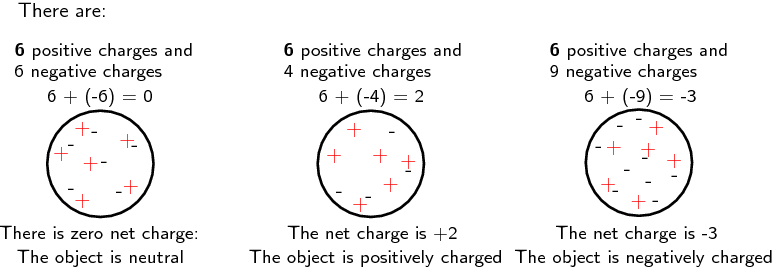# Electric Charge

Electric charge refers to the charge an object has due to the number of protons and electrons present in the atoms it is made up of.

There are two types of electric charge - positive and negative charge. These have been arbitrarily named as positive and negative by scientists, but could just as easily been called red and blue. In other words, the name does not matter; it is how the charges behave that is important.

The electric charge of objects originates from the atoms they are made up of. Atoms are made up of neutral neutrons, positively charged protons, and negatively charged electrons. The charge of an atom is determined by the change in the number of electrons relative to the number of protons. If there are more electrons than protons (i.e. a gain of electrons), then the atom will be negatively charged. Conversely, if there are more protons than electrons (i.e. a loss of electrons), then the atom will be positively charged. If the number of protons is equal to the number of electrons then the atom is neutral and no net charge exists. Remember, that only electrons can be lost or gained, not protons.

All objects (matter) are made up of atoms, and thus, all objects have charge. This charge can be positive or negative, or an object can be neutral. The diagram below provides examples of how the number of electrons relative to the number of protons determines the charge of an object.Positive and negative charges exert a force on each other, known as the electrostatic force. Like charges repel one another and unlike charges attract. In other words, if a positively charged object comes into contact with another positively charged object, then they will repel one another. The same holds true for two negatively charged objects. Conversely, if a positively charged object comes into contact with a negatively charged object, the two objects will be attracted to one another. The strength of the electrostatic force (attraction or repulsion) is determined by the distance between the two objects. The closer together the objects are, the stronger the force. The further apart the objects are, the weaker the force.

Charge cannot be created or destroyed - it can only be transferred from one object to another. This is known as the principle of conservation of charge.

Charged can be quantified or given a value. This value is based on the elementary charge or basic unit of charge of one electron. This is represented by qe, which is equal to 1.6 x 10-19 C. The value of charge (Q) is measured in coulombs (C) and for multiple electrons can be represented by Q = nqe.

In conclusion, there are two types of charge, positive and negative. Like charges repel and unlike charges attract. Charge is conserved and can be quantified.